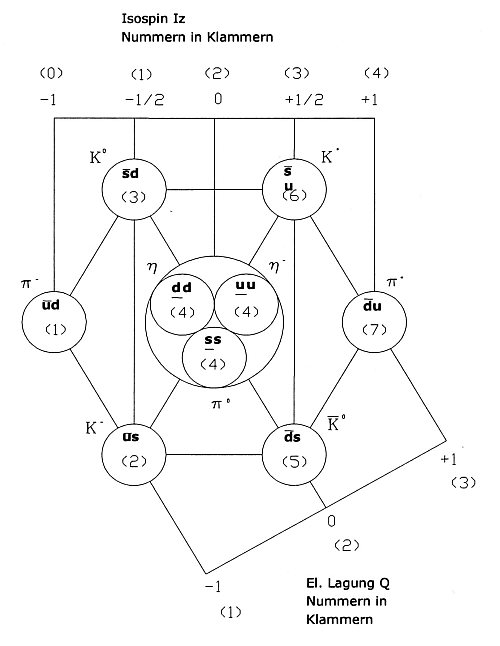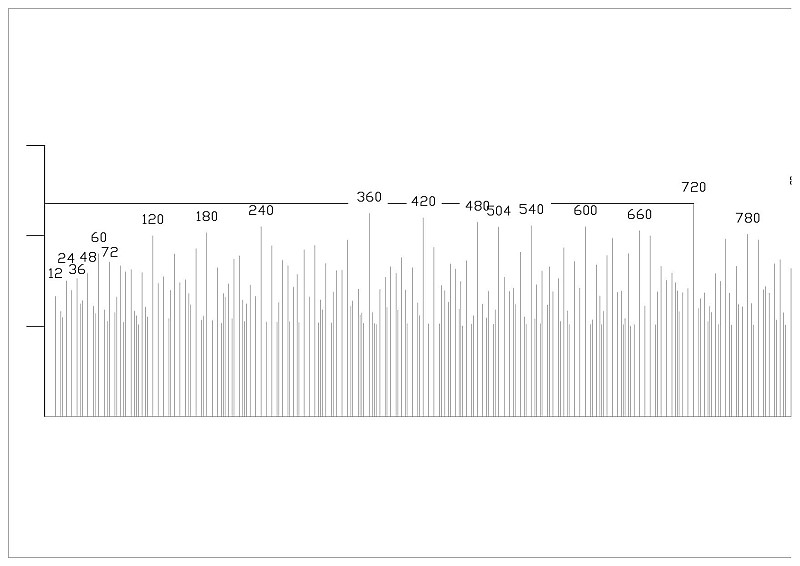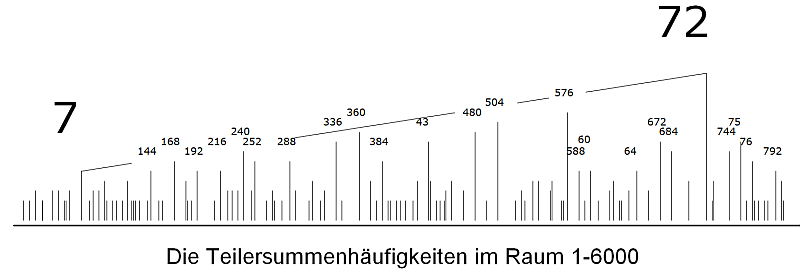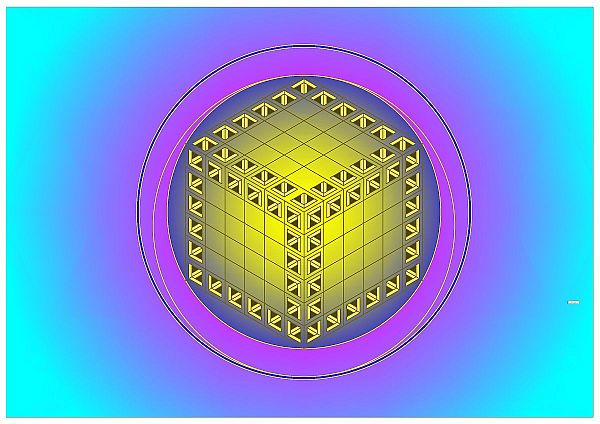# Perfect Numbers

A contribution of Willibald Limmbrunner

A natural number n is called a perfect number
if it is equal to the sum of all its (positive) divisors
except itself.
Wikipedia / Perfect Number

The simplest example: The number six has three divisors 1, 2 and 3. The sum of these divisors is 1 + 2 + 3 = 6. The number six is ​​thus a perfect number. The sum of the divisors of a number n is called Sigma σ * (n). So it’s like that: σ * (6) = 6. In words, the divisor sum of six is ​​six.

σ (n): Often, as a divisor of a number, the number itself is also added, then the divisor sum is called σ (n). σ (n) = 1 + 2 + 3 + 6 = 12. It is especially nice to put the divisors into pairs: 2 x 3 = 1 x 6 = 6

Abundant Numbers: Not every number is a perfect number. σ * (12) = 1 + 2 + 3 + 4 + 6 = 16. Here the divisor sum is larger than the number itself. Such numbers are called Abundant Numbers.

Deficient numbers: On the other hand, σ * (8) = 1 + 2 + 4 = 7. Numbers whose divisor sum is smaller than the number itself are called Deficient Numbers.

The next perfect number is σ * ​​(28) = 1 + 2 + 4 + 7 + 14 = 28. The next perfect number is 496. ->

Other regularities of perfect numbers

Harmonic aspects of perfect numbers.

An overtone is particularly catchy when it has as many partials as possible. The fifth overtone of a fundamental sound sounds strange, or new and unfamiliar, if one plays the notes individually. The frequencies 100 Hz, 200 Hz, 300 Hz and 400 Hz sound particularly harmonious and catchy. The frequency ratios octaves (1: 2: 4) and fifths (2: 3) are found. The fifth overtone with 500 Hz sounds new, compared to the previous unfamiliar. The big third with the frequency ratio 4: 5 is new, since the divisibility of the number five is small. Five is also a prime number. The divisor sum σ * (5) = 1.

Musically, the intervals octave and fifth move in the pentatonic. In terms of music history, the occurrence of the Grand Third, for example with the Renaissance, is a turning point. The thirds are the beginning of the music of the fifth-thirds system in which we still make music today. In todays music septims are not natural septims with the frequency ratios 6: 7: 8, but they are integrated in the fifth-thirds system, for example as a diatonic seventh H in the principal triad GHD (major third + minor third, frequency ratio 4: 5: 6).

The first perfect number six plays a prominent role in the harmony of Hans Kayser. He named the first intervals of frequency ratios 1: 2: 3: 4: 5: 6, Senarius. Kayser said it was the harmonic-sounding intervals, while the Septime 6: 7 was disharmonious. However, listening to 6: 7: 8, or about 5: 6: 7, sounds harmonious as well (harmony theory after Johannes Kotschy).

The Senarius (1: 2: 3: 4: 5: 6), however, has other qualities. It contains all harmonic-sounding and constituent intervals of the fifth-thirds system. The frequency proportions 1: 2: 3: 4, written separately 1: 1, 1: 2, 2: 3, 3: 4, brought to common denominator (6:12, 8:12, 9:12, 12:12) and ordered, the Harmonia perfecta maxima (6,8,9,12), the tone sequences C – F – G – C ‚, (Tonic Dominant Subdominant Tonics), the base cadence. The triad over the tone levels (4: 5: 6 frequency proportion) gives the complete diatonic scale.

The hexagonal geometry of Senarius as a basic structure of the world.
The regular polygon with seven vertices can not be constructed with a compass and a ruler, so the angular functions of the seven-part circle are not rational but transcendental numbers. In this respect, the seven forms a natural boundary in the symmetry considerations of the circle. This corresponds to the quintet system with its constituent intervals (1: 2: 3: 4: 5: 6), without this being intended by the music theorists. Our music, which we operate from about the Enlightenment, moves in this context below the seven. The hexagon holds the seven in its midst, in a way unrevealed, hidden.(Figure from: Limbrunner, Willibald; Zahl Seele Kosmos; Synergiea Verlag; 2010; Abb. 63.)

Indeed, even quantum physics discovered, with the symmetries of the quarks, the hexagonal structure as a fundamental symmetry of the heavy particles. So those particles, which i.a. form the nucleus. The above figure shows the symmetries of baryons and Mesons.Divisor sums up to 720, see figure above: The multiples of twelve (12, 24, 36, 48 …) have very large divisor sums σ *. The number twelve possesses especially many divisors (1,2,3,4,6). Only with the number 60 and its multiples, the divisor sums are again considerably larger. 60 is the first multiple of 12 which is also divisible by 5. 60 is a particularly well divisible number. With the number 60, the triads of the fifth-thirds system occur: (1/4: 1/5: 1/6 = 15/60: 12:60: 10/60), KgV {4, 5, 6} = 60. The number 720 = 1 x 2 x 3 x 4 x 5 x 6 again dominates all previous divisor sums.

The number 5040: The number 5040 = 1 x 2 x 3 x 4 x 5 x 6 x 7 occurring in Plato’s state (Politeia). The optimal number of people living in an ideal state surpasses all previous ones. With this number, the seven is added.

Number and Harmony: So perfect and abundant numbers, which have many dividers, are especially catchy as overtones, contain many partials, which resonate and resonate strongly with their partials. In this way one can see how elementary number theory and harmony interact. The quotation from the hearings of Pythagoras shows its concrete meaning in this example:

What is the Wisest? Number.

The number 72: Not all numbers are the same, each has its peculiarity. Outstanding among the divisor sums is the number 72. In the number range 1 to 6000, the number 72 has the largest subtotal frequency. Ie. it is most common as a divisor in the numbers 1 to 6000 before. Example:
30 = 1×30, 2×15, 3×10, 5×6         σ (30) = 72
46 = 1×46, 2×23                          σ (30) = 72
30 and 46 have the same splitter sum 72.
The number itself is included as a splitter σ(n).The number 72 has the divisors 1, 2, 3, 4, 6, 8, 9, 12, 18, 24, 36, 72. The bold numbers contain both forms of the tetrahedra sacred to the Pythagoreans (1,2,3, 4), (6,8,9,12). The latter form was called „Harmonia perfecta maxima“ by the Roman philosopher and writer Boetius.
72.